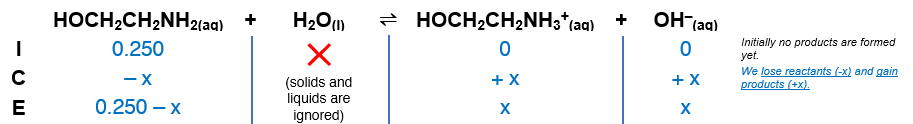# Problem: What is the pH of 0.25 M ethanolamine, HOCH2CH2NH2, (Kb=3.2x10-5)?

###### FREE Expert Solution

HOCH2CH2NH2(aq) + H2O(l)  HOCH2CH2NH3+(aq) + OH(aq); Kb = 3.2 × 10–5

Step 1Construct an ICE table.The Kb expression for HOCH2CH2NH2 is:

$\overline{){{\mathbf{K}}}_{{\mathbf{b}}}{\mathbf{=}}\frac{\mathbf{products}}{\mathbf{reactant}}{\mathbf{=}}\frac{\mathbf{\left[}{\mathbf{HOCH}}_{\mathbf{2}}{\mathbf{CH}}_{\mathbf{2}}{{\mathbf{NH}}_{\mathbf{3}}}^{\mathbf{+}}\mathbf{\right]}\mathbf{\left[}{\mathbf{OH}}^{\mathbf{-}}\mathbf{\right]}}{\left[{\mathrm{HOCH}}_{2}{\mathrm{CH}}_{2}{\mathrm{NH}}_{2}\right]}}$###### Problem Details

What is the pH of 0.25 M ethanolamine, HOCH2CH2NH2, (Kb=3.2x10-5)?